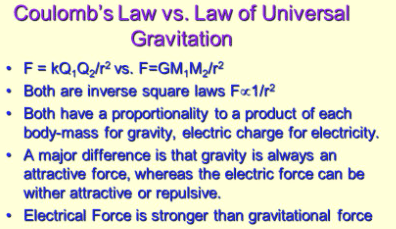Electricity & Megnetism

# coulomb’s and newton’s gravitational law: differences and similaritiesCoulomb’s law Vs Gravitational law The difference between Coulomb’s law and gravitational law is provided here. If you want to learn Brief differences b/w law of Electrostatic and Universal law of gravitation or gravitational law, then you are at the right place.

## Comparison of Coulomb’s law and Newton’s law of gravitation

The electrical force between two charges is directly proportional to the product of their charges and inversely proportional to the square of the distance between them: It is expressedThe gravitational force between two masses is directly proportional to the product of their masses and inversely proportional to the square of the distance between them:### Differences among the electrical and gravitational force

• Electrical force is might be attractive as well as repulsive, while the gravitational force is only attractive.
• The electrostatic force is medium dependent and can be shielded while the gravitational force lacks this property.
• The value of the gravitational constant is very small while the electrical constant is very large. It is because of the fact that the gravitational force is very weak as compared to electrical force.
• In using the law of gravitation, we define mass ‘m’ from F=ma, then determine ‘G’ by applying gravitational law to known masses. While in the case of coulomb’s law, we define ‘k’ for a particular value and then determine ‘q’ by applying coulomb’s law.

Related Topics:
Coulomb’s law
Newton’s law of gravitation